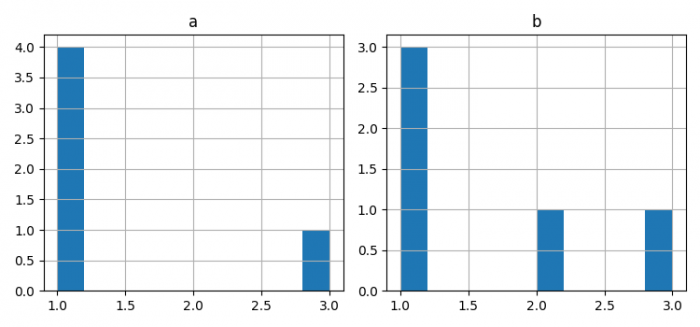# How to plot two histograms side by side using Matplotlib?

To plot two histograms side by side using matplotlib, we can take the following steps −

• Set the figure size and adjust the padding between and around the subplots.

• Make two dataframes, df1 and df2, of two-dimensional, size-mutable, potentially heterogeneous tabular data.

• Create a figure and a set of subplots.

• Make a histogram of the DataFrame's, df1 and df2.

• To display the figure, use show() method.

## Example

from matplotlib import pyplot as plt
import pandas as pd

plt.rcParams["figure.figsize"] = [7.50, 3.50]
plt.rcParams["figure.autolayout"] = True

df1 = pd.DataFrame(dict(a=[1, 1, 1, 1, 3]))
df2 = pd.DataFrame(dict(b=[1, 1, 2, 1, 3]))

fig, axes = plt.subplots(1, 2)

df1.hist('a', ax=axes)
df2.hist('b', ax=axes)

plt.show()

## Output